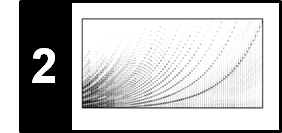# Chapter 2: Fourier Analysis of Signals

The Fourier transform is perhaps the most fundamental tool in signal processing. Chapter 2 of [Müller, FMP, Springer 2015] approaches Fourier analysis from various perspectives and discusses their most important properties in the context of audio signal processing. In particular, the notion of a spectrogram, which yields a time–frequency representation of an audio signal, is introduced.

2.1 The Fourier Transform in a Nutshell
2.2 Signals and Signal Spaces
2.3 Fourier Transform
2.4 Discrete Fourier Transform (DFT)
2.5 Short-Time Fourier Transform (STFT)
2.6 Further Notes

## Notebooks¶

 Topic Relation to [Müller, FMP, Springer 2015] & Description HTML IPYNB Complex Numbers [Section 2.2.3.1, Exercise 2.1]Absolute value; angle; polar representation; conjugation; inverse; arrow plot; polar coordinate plot [html] [ipynb] Exponential Function [Section 2.3.2.1, Exercise 2.15]Power series; Euler's formula; root of unity [html] [ipynb] Discrete Fourier Transform (DFT) [Section 2.1.3, Section 2.4]Inner product; DFT matrix; fast Fourier transform (FFT); runtime experiments [html] [ipynb] DFT: Phase [Section 2.3.2]Exponential function; polar coordinates; complex Fourier coefficient; phase; optimality property [html] [ipynb] Discrete Short-Time Fourier Transform (STFT) [Section 2.1.4, 2.5.3]Time localization; spectrogram; physical interpretation; interactive plot [html] [ipynb] STFT: Influence of Window Function [Section 2.5]Window type; window size [html] [ipynb] STFT: Padding [Section 2.1.4, Section 2.5.3]Padding variants; edge phenomena; interactive plot [html] [ipynb] STFT: Frequency Grid Density [Section 2.1.4]DFT frequency grid; zero padding; increasing density [html] [ipynb] STFT: Frequency Interpolation [Section 2.1.4, Section 3.1.1]Linear interpolation; cubic interpolation; log-frequency STFT; cents [html] [ipynb] STFT: Inverse [Section 2.4.2, Section 8.1.2.1]DFT; inverse DFT; window function; overlap–add technique; artition of unity [html] [ipynb] STFT: Conventions and Implementations [Section 2.1.4, 2.5.3]Time axis convention; centered windowing; frequency conversion; implementation issues [html] [ipynb] Digital Signals: Sampling [Section 2.2.2.1, Exercise 2.28]Equidistant sampling; aliasing; signal reconstruction; sinc function; sampling theorem [html] [ipynb] Digital Signals: Quantization [Section 2.2.2.2, Exercise 2.9]Uniform quantization; quantization error [html] [ipynb] Interference and Beating [Section 2.3.3.1, Exercise 2.19]Constructive interference; destructive interference; chirp; sweep [html] [ipynb]